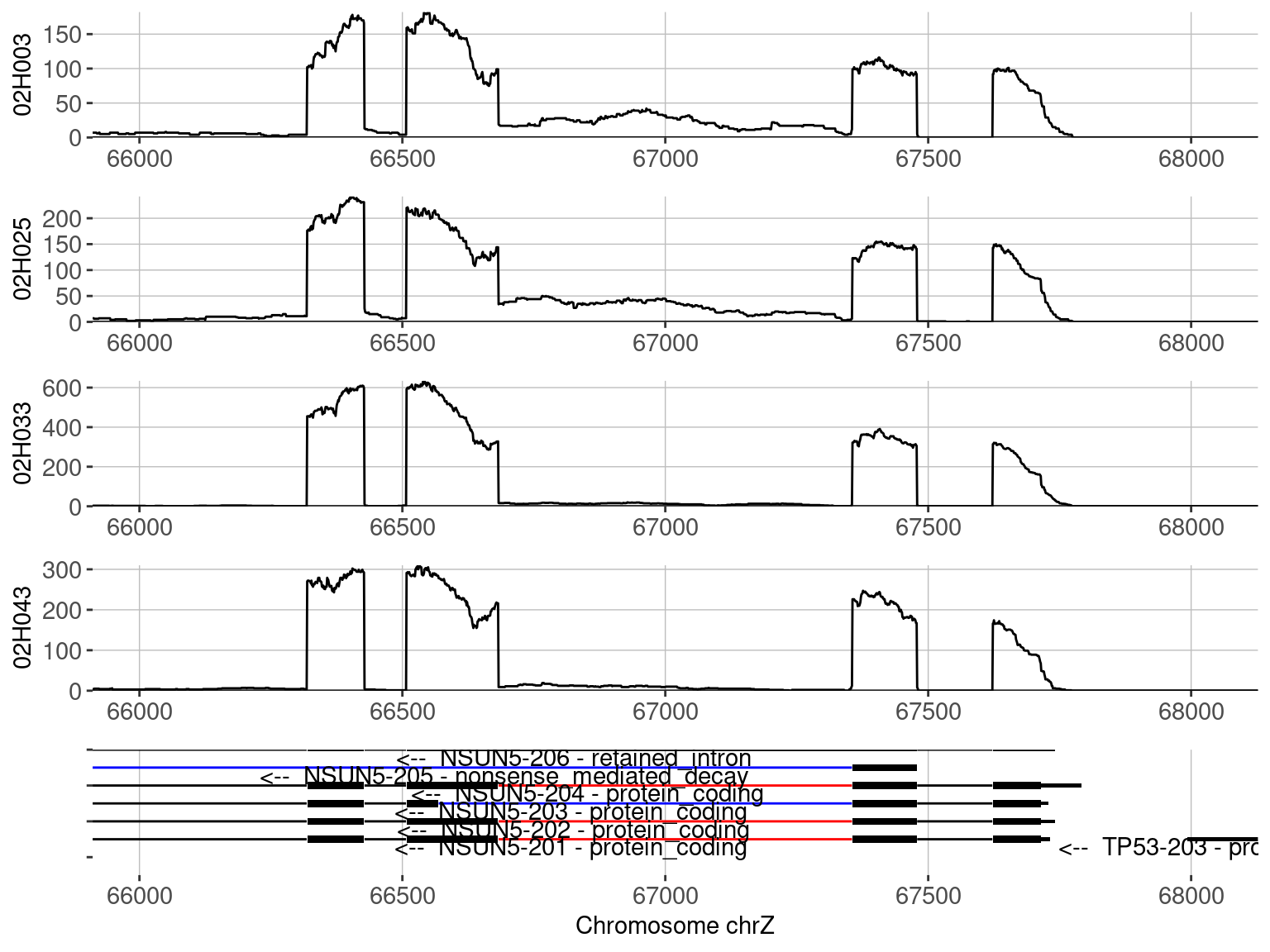# (1) Installation and Quick-Start

This section provides instructions for installation and a quick working example to demonstrate the important functions of NxtIRF. NxtIRFcore is the command line utility for NxtIRF.

For detailed explanations of each step shown here, refer to chapter 2: “Explaining the NxtIRF workflow” in this vignette. For a list of ready-made “recipes” for typical-use NxtIRF in real datasets, refer to chapter 3: “NxtIRF cookbook”

### Installation

To install NxtIRFcore, start R (version “4.1”) and enter:

``````if (!requireNamespace("BiocManager", quietly = TRUE))
install.packages("BiocManager")

BiocManager::install("NxtIRFcore")``````

(Optional) For MacOS users, make sure OpenMP libraries are installed correctly. We recommend users follow this guide, but the quickest way to get started is to install `libomp` via brew:

``brew install libomp``

``````library(NxtIRFcore)

### Building the NxtIRF reference

A NxtIRF reference requires a genome FASTA file (containing genome nucleotide sequences) and a gene annotation GTF file (preferably from Ensembl or Gencode).

NxtIRF provides an example genome and gene annotation which can be accessed via the NxtIRFdata package installed with NxtIRF:

``````# Provides the path to the example genome:
chrZ_genome()
#>  "/home/biocbuild/bbs-3.15-bioc/R/library/NxtIRFdata/extdata/genome.fa"

# Provides the path to the example gene annotation:
chrZ_gtf()
#>  "/home/biocbuild/bbs-3.15-bioc/R/library/NxtIRFdata/extdata/transcripts.gtf"``````

Using these two files, we construct a NxtIRF reference as follows:

``````ref_path = file.path(tempdir(), "Reference")
BuildReference(
reference_path = ref_path,
fasta = chrZ_genome(),
gtf = chrZ_gtf()
)``````

### Running IRFinder

NxtIRF provides an example set of 6 BAM files to demonstrate its use via this vignette.

Firstly, retrieve the BAM files from ExperimentHub using the NxtIRF helper function `NxtIRF_example_bams()`. This makes a copy of the BAM files to the temporary directory:

``````bams = NxtIRF_example_bams()
bams
#>   sample                       path
#> 1 02H003 /tmp/Rtmp4oyPLE/02H003.bam
#> 2 02H025 /tmp/Rtmp4oyPLE/02H025.bam
#> 3 02H026 /tmp/Rtmp4oyPLE/02H026.bam
#> 4 02H033 /tmp/Rtmp4oyPLE/02H033.bam
#> 5 02H043 /tmp/Rtmp4oyPLE/02H043.bam
#> 6 02H046 /tmp/Rtmp4oyPLE/02H046.bam``````

Finally, run NxtIRF/IRFinder as follows:

``````irf_path = file.path(tempdir(), "IRFinder_output")
IRFinder(
bamfiles = bams\$path,
sample_names = bams\$sample,
reference_path = ref_path,
output_path = irf_path
)``````

### Collate individual IRFinder runs to build a NxtIRF Experiment

First, collate the IRFinder output files using the helper function `Find_IRFinder_Output()`

``expr = Find_IRFinder_Output(irf_path)``

This creates a 3-column data frame with sample name, IRFinder gzipped text output, and COV files. Compile these output files into a single experiment:

``````nxtirf_path = file.path(tempdir(), "NxtIRF_output")
CollateData(
Experiment = expr,
reference_path = ref_path,
output_path = nxtirf_path
)``````

### Importing the collated data as a NxtSE object:

The `NxtSE` is a data structure that inherits `SummarizedExperiment`

``se = MakeSE(nxtirf_path)``

### Set some experimental conditions:

``````colData(se)\$condition = rep(c("A", "B"), each = 3)
colData(se)\$batch = rep(c("K", "L", "M"), 2)``````

### Perform differential alternative splicing

The code below will contrast condition:B in respect to condition:A

``````# Requires limma to be installed:
require("limma")
res_limma = limma_ASE(
se = se,
test_factor = "condition",
test_nom = "B",
test_denom = "A",
)

# Requires DESeq2 to be installed:
require("DESeq2")
res_deseq = DESeq_ASE(
se = se,
test_factor = "condition",
test_nom = "B",
test_denom = "A",
)

# Requires DoubleExpSeq to be installed:
require("DoubleExpSeq")
res_DES = DoubleExpSeq_ASE(
se = se,
test_factor = "condition",
test_nom = "B",
test_denom = "A",
)``````

### Visualise a Coverage plot of a differential IR event:

Filter by visibly-different events:

``res_limma.filtered = subset(res_limma, abs(AvgPSI_A - AvgPSI_B) > 0.05)``

Plot individual samples:

``````p = Plot_Coverage(
se = se,
Event = res_limma.filtered\$EventName,
tracks = colnames(se)[c(1,2,4,5)],
)
#> Warning: `gather_()` was deprecated in tidyr 1.2.0.
#> This warning is displayed once every 8 hours.
#> Call `lifecycle::last_lifecycle_warnings()` to see where this warning was generated.
as_egg_ggplot(p)``````Display the plotly interactive version of the coverage plot (not shown here)

``````# Running this will display an interactive plot
p\$final_plot``````

Plot by condition:

``````p = Plot_Coverage(
se = se,
Event = res_limma.filtered\$EventName,
tracks = c("A", "B"),
condition = "condition",
stack_tracks = TRUE,
t_test = TRUE,
)
as_egg_ggplot(p)
#> Warning: Removed 270 row(s) containing missing values (geom_path).``````